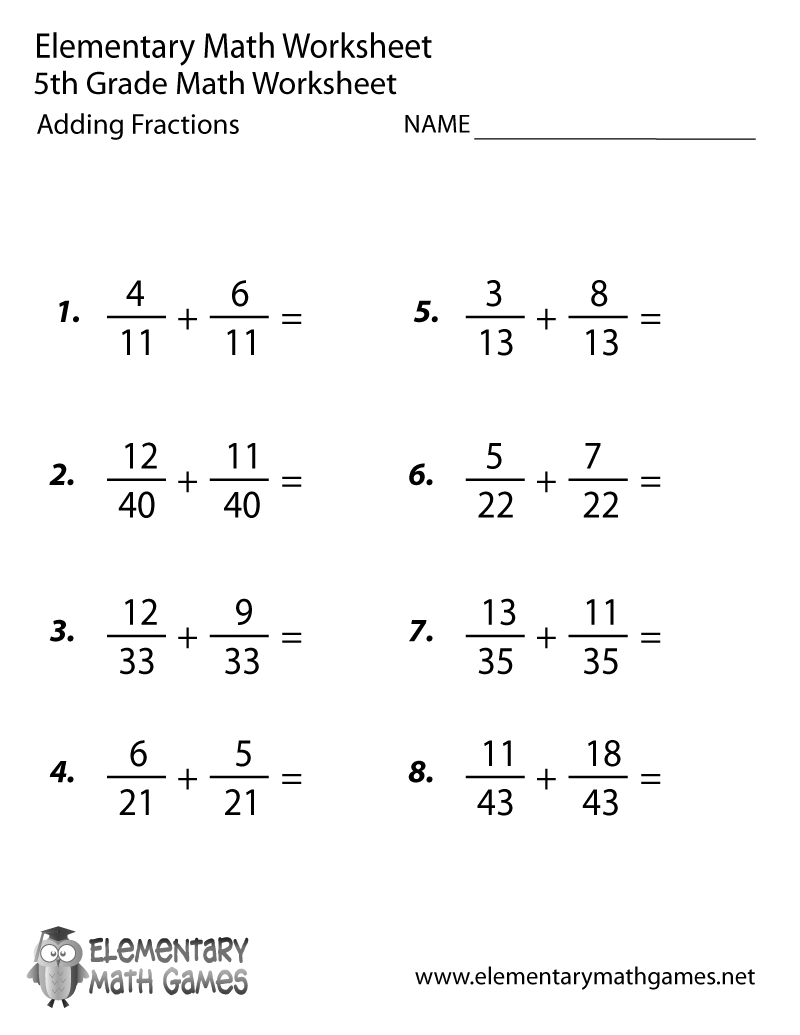## Learn and Practice Adding Fractions

This fifth grade math worksheet has 8 fraction addition problems for kids to solve. Fifth graders have to add fractions that have the same denominators.

Here are the two versions of this free fifth grade math worksheet: